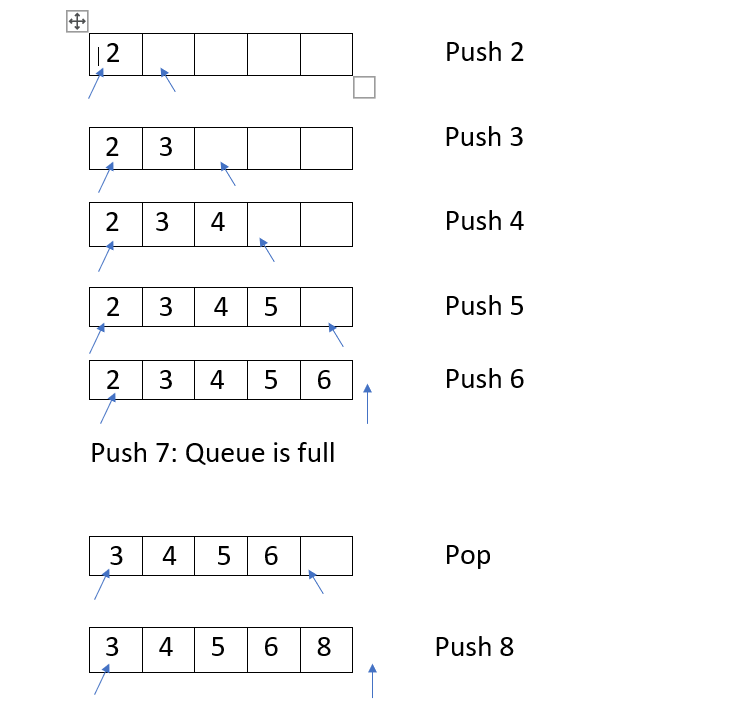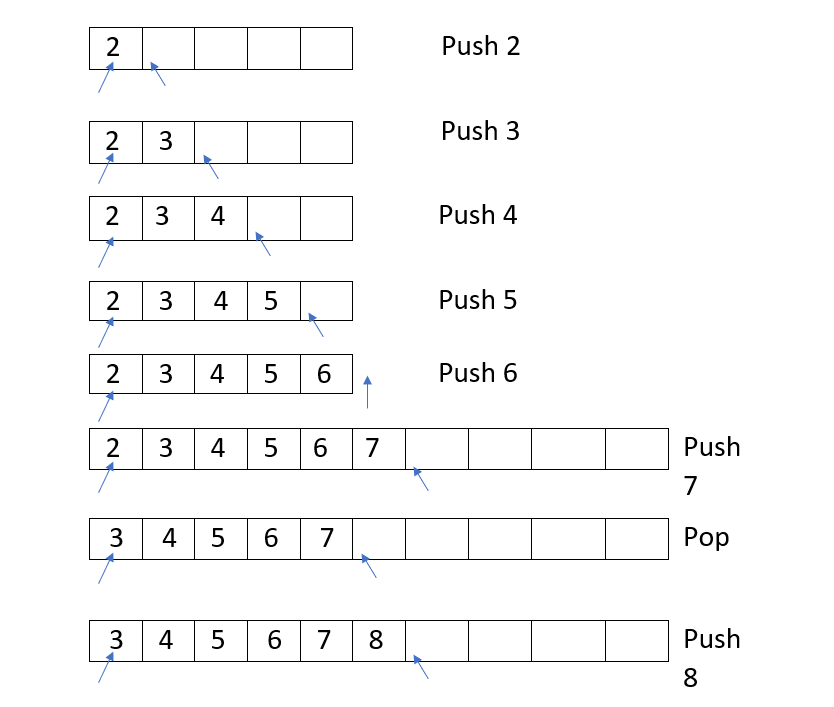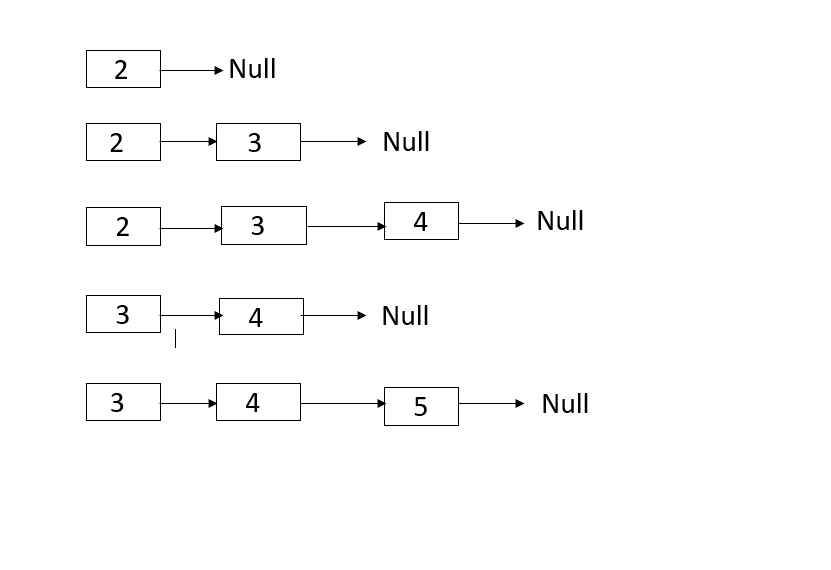# Queue using C++ classes [using OOP and Template] [no STL]We have explained different ways to implement Queue data structure in C++ using OOP (Object Oriented Programming) concepts and Templates in C++. We have not used STL C++. We have presented the basics of Queue and Template in C++ as well.

Table of content:

1. Basics of Queue and Templates in C++
2. Implementing Queue Using Array in C++
3. Implementing Queue using Linked List in C++
4. Application of Queue
5. Question

We will get started now.

# Basics of Queue and Templates in C++

A queue is a linear data structure. It works on the principle of First In First Out (FIFO) where we can insert data from one end called "rear" and can remove data from other end called "front" or you can say in this data structure whichever element is being inserted first will be removed first. A real world example of queue can be a single-lane one-way road,where the vehicle enters first,exits first.

To deal with different datatype in queue we can make use of a very powerful tool of c++,templates.Let's first learn some basic concepts about templates.

## Templates

Templates are used to avoid writing code for each datatype separately.Instead we can write a generic code by simply passing datatype as a parameter.

### Function Templates

``````#include<iostream>
using namespace std;

template<typename T>
T func(T data){
return data;
}

template<typename T>   /* Here templates needs to be defined once again */
T MYmin(T data1,T data2){
if(data1>data2){
return data2;
}else{
return data1;
}
}

int main(){
// T ans = func<int>(7);   /* T will give compile error here since we can't use Template at the time of memory allocation. We need to specify datatype(generic or custom) of T. */
cout<<func<int>(7)<<endl;    //output: 7
cout<<func<char>('A')<<endl;   //output: A
cout<<MYmin<int>(7,10)<<endl;  //output: 7
}
``````

### Class Templates

It is mostly used during the implementation of custom classes for e.g. Stacks,Queue etc.

`````` #include<iostream>
using namespace std;

template<typename T>
class base{
T first;
public:
void setFirst(T first){
this->first=first;
}
T getFirst(){
return first;
}
};

int main(){
base<int> b1;
b1.setFirst(5);
cout<<b1.getFirst()<<endl;  //output: 5

base<char> b2;
b2.setFirst('a');
cout<<b2.getFirst()<<endl;   //output: a
}
``````

Queue can be implemented using :

• Array

# Implementing Queue Using Array in C++

Firstly we will see a very simple implementation of queue which uses static array to store data. To dynamically incorporate unlimited data (upto allocation space available) we would need dynamic array.

Basic Operations in queue :

• Push() : This operation helps us to insert element in the queue.It doesn't return anything.
• Pop() : This helps us to remove an element from queue.It returns the element which is being removed.

There are some additional operations with which we are going to deal in queue are:

• isEmpty() : Tells us if queue is empty.It returns true when queue will be empty.
• getSize() : It provides us the number of elements which are there in queue.
• front() : It returns the element which is present at the top of the queue.
``````#include<iostream>
using namespace std;

template<typename T>
class QueueUsingArray{
T* data;
int frontIndex;    // It stores the index of an element which is present at the top of a queue.
int rearIndex;     // It tells us the next position in the array where the element is to inserted.
int maxSize;      // It stores the total capacity of an array.
int size;         // It stores the number of elements which are being present in the array.

public:
QueueUsingArray(int size){
maxSize = size;
data = new T[maxSize];
frontIndex = -1;
rearIndex = 0;
this->size = 0;
}

int getSize(){
return size;
}

bool isEmpty(){
return size == 0;
}

T front(){
if(frontIndex == -1){
cout<<"Queue is empty "<<endl;
return 0;
}
return data[frontIndex];
}

void push(T elem){
if(size == maxSize){
cout<<"Queue is full "<<endl;
return;
}

if(frontIndex == -1){
frontIndex = 0;
}

data[rearIndex] = elem;
rearIndex++;
size++;
}

T pop(){
if(frontIndex == -1){
cout<<"Queue is empty "<<endl;
return 0;
}
T temp = data[frontIndex];
for (int i = 0; i <size; i++) {
data[i] = data[i + 1];
}
rearIndex--;
size--;
if(size == 0){
frontIndex = -1;
rearIndex = 0;
}
return temp;
}
};

int main(){
QueueUsingArray<int> q1(5);
q1.push(2);
q1.push(3);
q1.push(4);
q1.push(5);
q1.push(6);
q1.push(7);
cout<<"Element which is being removed "<<q1.pop()<<endl;
q1.push(8);

QueueUsingArray<char> q2(5);
q2.push('a');
q2.push('b');
q2.push('c');
cout<<"Element which is being removed "<<q2.pop()<<endl;
}
``````Above method has a limitation that the size of the queue will be fixed.Size will become equal to the one that the user has defined in the beginning,now it can't be changed but we can overcome this constraint by just changing push operation as follows:

`````` void push(T elem){
if(size == maxSize){
T* newdata=new T[2*maxSize];
for(int i=0;i<maxSize;i++){
newdata[i]=data[i];
}
delete []data;
data=newdata;
maxSize=maxSize*2;
}

if(frontIndex == -1){
frontIndex = 0;
}

data[rearIndex] = elem;
rearIndex++;
size++;
}
``````In this implementation of push operation,we are just making a slight change that is if anytime queue gets full we are increasing it's size by dynamically allocated an array.

In the array implementation of a queue :

• Time Complexity of a Push Operation : O(1)
• Time Complexity of a Pop Operation : O(maxSize)

# Implementing Queue using Linked List in C++

Implementation of Queue using Linked List in C++ is:

``````#include<iostream>
using namespace std;

template <typename T>
class Node {
public :
T data;
Node<T> *next;

Node(T data) {
this -> data = data;
next = NULL;
}
};

template<typename T>
class QueueUsingLL {
Node<T> *tail;
int size;

public :

QueueUsingLL() {
tail=NULL;
size=0;
}

void push(T data) {
Node<T> *newnode=new Node<T>(data);
if(tail==NULL){
tail=newnode;
}else{
tail->next=newnode;
tail=tail->next;
}
size++;
}

int getSize() {
return size;
}

bool isEmpty() {
return size==0;
}

T pop() {
// Return 0 if queue is empty
return 0;
}
delete help;
size--;
if(size==0){
tail=NULL;
}
return ans;
}

T front()  {
// Return 0 if queue is empty
return 0;
}
}
};
int main(){
QueueUsingLL<int> q;
q.push(2);
q.push(3);
q.push(4);
cout<<q.pop()<<endl;
q.push(5);
}
``````In the linked-list implementation of a queue,there is no issue of fixed size, in this case store as many elements in the queue as we want.

• Time Complexity of Push Operation : O(1)
• Time Complexity of Pop Operation : O(1)

# Application of Queue

• Various CPU Scheduling Algorithms are implemented using queue data structure like Round Robin algorithm.
• In graph data structure,Breadth First Algorithm(in which each node first try to explore it's neighbour nodes and then move on to the next level node) also uses queue data structure.

# Question

Queue is initialized with size=3 and following operations are being performed(Assuming queue is implemented using array and there will be no dynamic allocation of array) Push(20),Push(25),Push(28),Push(99),Pop(),Pop(),Pop().Now once again Pop() will be called then what will be the output ?

Options are :

• 28
• 99
• Queue is empty
• None of these

With this article at OpenGenus, you must have a strong idea of different ways to implement Queue in C++.